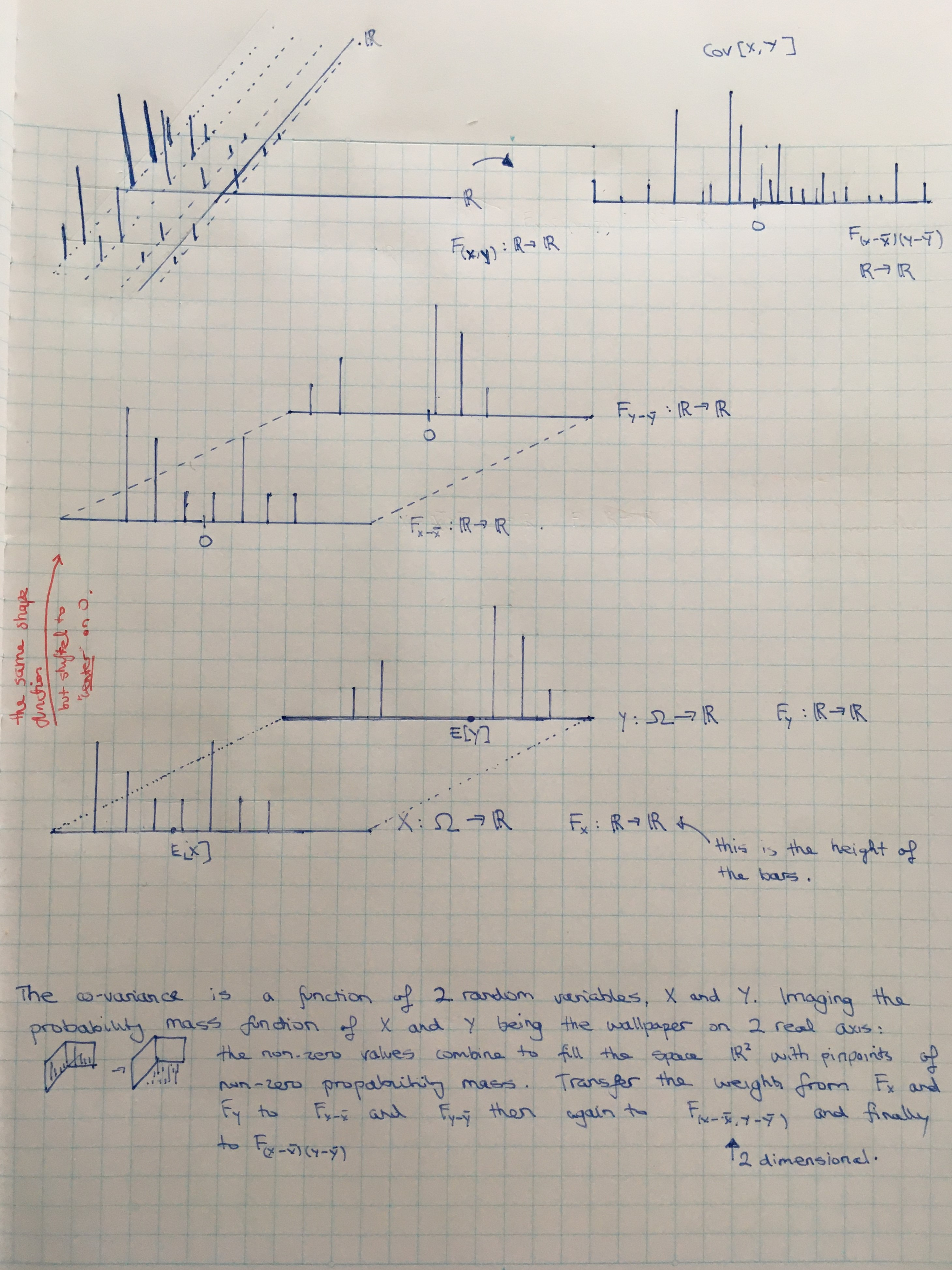Show Question
Math and science::INF ML AI

# Covariance matrix

Let $$X$$ and $$Y$$ be two random variables. The covariance between $$X$$ and $$Y$$ is defined as:

\begin{aligned} Cov[X,Y] &:= E[(X-E[X])(Y-E[Y])] \\ &= E[XY] - E[X]E[Y] \end{aligned}

Let the vector $$Z$$ be defined like so: $$Z := \begin{bmatrix} X \\ Y\end{bmatrix}$$. Thus, $$Z$$ is a vector of random variables.

The covariance matrix for $$Z$$ is defined as:

\begin{aligned} Cov[Z] &:= E[(Z - E[Z])(Z - E[Z])^T] \\ &= \begin{bmatrix} Var(X) & Cov(X, Y) \\ Cov(X, Y) & Var(Y) \end{bmatrix} \\ \end{aligned}

Where the expectation is an elementwise operation. The covariance matrix is a result of a matrix multiplication of two vector-like matrices, which produces a 2x2 matrix. (Yes, it is valid!).

### Matrix interpretation

An intepretation of such a 2x1*1x2 matrix multiplication is:

\begin{aligned} \begin{bmatrix} A \\ B \end{bmatrix} \begin{bmatrix}C & D\end{bmatrix} &= \begin{bmatrix}AC & AD \\ BC & BC \end{bmatrix} \\ &= \begin{bmatrix}C\begin{pmatrix}A \\ B\end{pmatrix} & D\begin{pmatrix}A \\ B \end{pmatrix} \end{bmatrix} \end{aligned}

The first matrix can be considered a transformation matrix which transforms a single dimension into 2 dimensions. $$A$$ is the factor by which the input scalar is multiplied by to produce the first output dimension; $$B$$ is the same quantity for the second output dimension. The matrix $$\begin{bmatrix} C & D\end{bmatrix}$$ can be considered a list of two separate scalars that will be transformed separately.

For the case of $$Z Z^T$$, if $$Z$$ has $$D$$ dimensions, then the output is D vectors combined horizontally into a matrix, where each vector is the original $$Z$$ multiplied by one of it's components.

For the 2 dimensional covariance matrix we have:

\begin{aligned} Cov[Z] &= E[ (Z - E[Z])(Z - E[Z])^T] \\ &= E\left[ \begin{bmatrix}X - \mu_X \\ Y - \mu_Y \end{bmatrix} \begin{bmatrix}X-\mu_X & Y-\mu_Y\end{bmatrix} \right] \\ &= E[\begin{bmatrix}(X - \mu_X) \begin{pmatrix} X - \mu_X \\ Y - \mu_Y\end{pmatrix} & (Y - \mu_Y) \begin{pmatrix} X - \mu_X \\ Y - \mu_Y\end{pmatrix} \end{bmatrix} ] \\ &= \begin{bmatrix} Cov(X, X) & Cov(Y, X) \\ Cov(X, Y) & Cov(Y, Y) \end{bmatrix} \\ &= \begin{bmatrix} Var(X) & Cov(X, Y) \\ Cov(X, Y) & Var(Y) \end{bmatrix} \end{aligned}

The covariance matrix is symmetric, like all matrixes of the form $$X X^T$$. Its diagonal is the variances of each random variable.

#### Random variable interpretation

Covariance is the expected value of the random variable $$Z = (X - \bar{X})(Y - \bar{Y})$$. Imagine the probability mass function of $$X$$ and $$Y$$, then $$X - \bar{X}$$ and $$Y - \bar{Y}$$, then the 2 dimensional $$(X - \bar{X}, Y- \bar{Y})$$, then finally the 1 dimensional $$Z$$. The covariance is a single value representing the expectation (product sum) of the value-probabilities of $$Z$$.